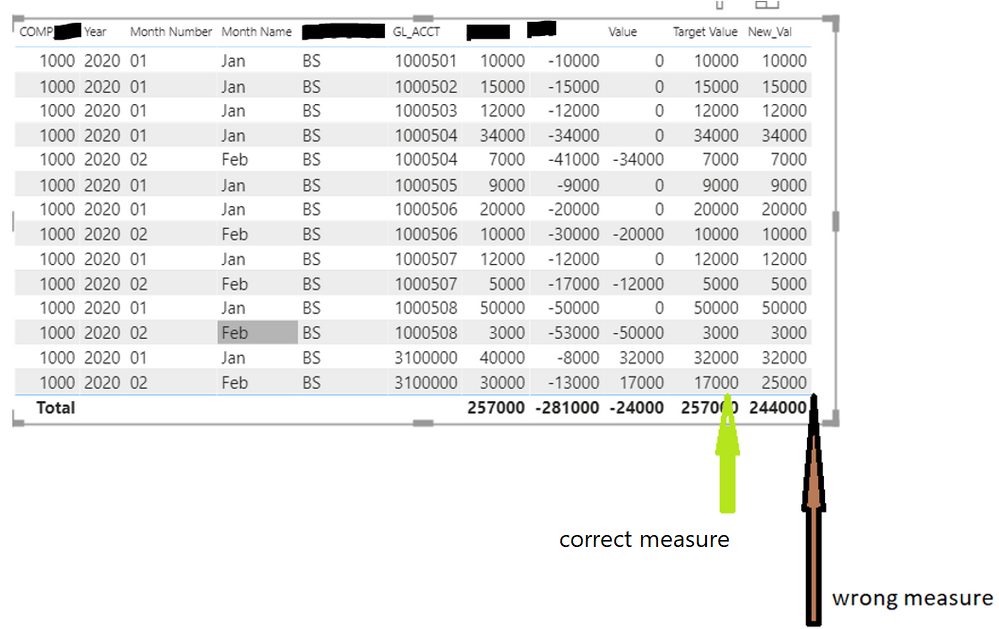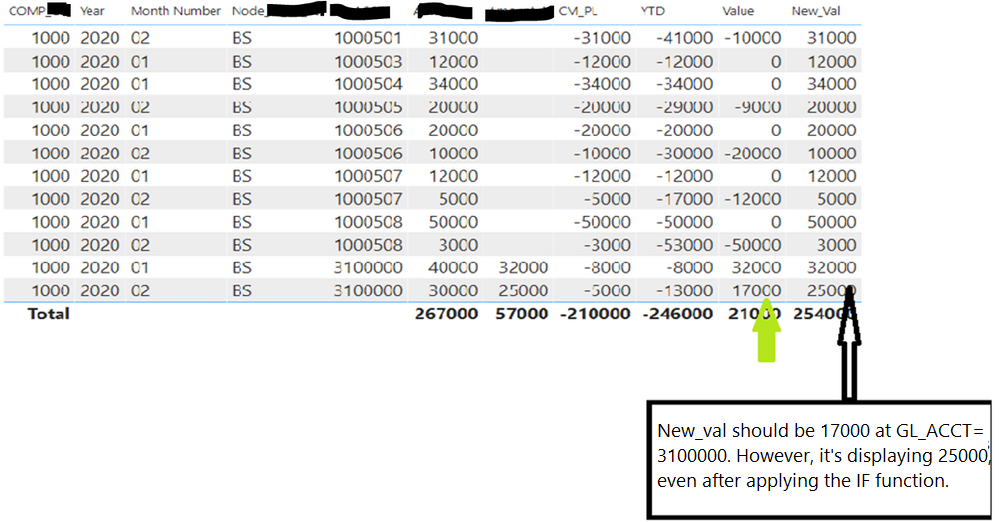cancel
Showing results for
Did you mean:## Custom Calculation Issue with Circular Dependency Fields

Issue: Custom calculations with circular dependency fields were not updating while performing YTD and IF DAX functions.

Requirement: Get updated value in the 'Target Value' column for the GL_ACCT 3100000, which is (Value +YTD_PL) for the Month 02. In the below screenshot, the number highlighted in red should be the output for the 'Target Value' column for 3100000 GL_ACCT.

Measures and Calculated Columns that were fetching incorrect results:

1.Value = SUM(data[Amount])+[YTD]

2.YTD = TOTALYTD(

SUM(data[CM_PL]),

'Calendar Table'[Date])

4.New_Val = IF(

data[GL_ACCT]=3100000,data[Value],data[Amount])Solution: Instead of creating the multiple measures, we have created variables in a single measure. The new measure 'Target value' shows the right values in the screenshot.

Target Value = var bc = SUM(data[Amount])

var ab = SUM(data[Amount]) + TOTALYTD(

SUM(data[CM_PL]),

'Calendar Table'[Date]

) Return IF(SUM(data[GL_ACCT])=3100000,ab,bc)Author: Arunkumar Karingali

Reviewer:Mounika Narayana Reddy